# Physics - Electromagnetism - Electromotive Force (EMF) and Internal resistance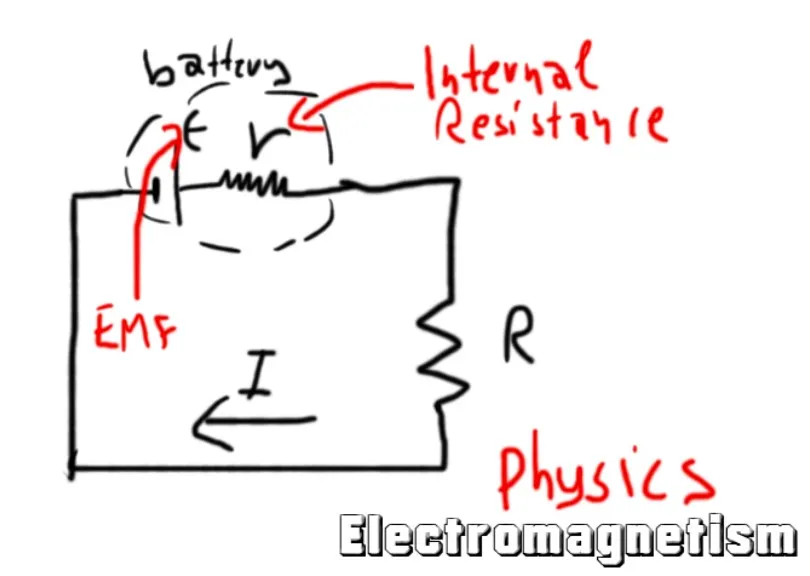Based on Farside figure 17 p 

## Introduction

Hello it's a me again Drifter Programming! Today we continue with Electromagnetism to get into Electromotive Force and Internal resistance. To understand this topic I highly recommend that you know a lot about current, resistance and potential already. So, without further do, let's get straight into it!

## Electromotive Force (EMF)

A constant electric current can flow through a conductor only when having a closed circuit and so cloced conductive path. This path cannot contain only ohmic resistors and capacitors, but should also have a potential difference from one end to the other. Only then the positive charges will move to the direction of the smallest potential energy (low) creating a constant flow. And so the energy source needs to be able of increasing the electric potential energy of a charge as it moves from the low to the high energy terminal. That's why all the circuits need to have a battery! 

Until now we saw that the positive charges tend to move in the direction high->low, which means that they want to decrease their potential energy. To continue on going in that direction there must be some force which pushes them in the opposite direction low->high for the time where the charges are inside of the battery. This exact force is defined as electromotive force (EMF). Any circuit with constant current flow must have a component that has a EMF! The term "force" in not decent, cause EMF is not a mechanical force measured in Newton's (N), but defined as the energy supply gained per unit charge (W/Q) and so kind of a potential. This is something that you should remember! 

The unit of electromotive force in SI is the same that potential has and so: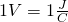but we use another symbol for it which is ε.

Note that the actual definition of them is different:

• Electromotive force is defined as the amount of energy supplied to one coulomb of charge (gain energy)
• Potential difference is the amount of energy used by one coulomb of charge to move from one point to another (loss energy)

An ideal EMF keeps the potential difference between it's terminals constant, independent of the current that flows through, cause it is the cause of the current. The EMF is defined equal to this exact potential difference or voltage V. EMF is the maximum voltage that the component/battery can attain.



From what we saw until now we can quite easily imagine that the inside of a EMF component looks like this: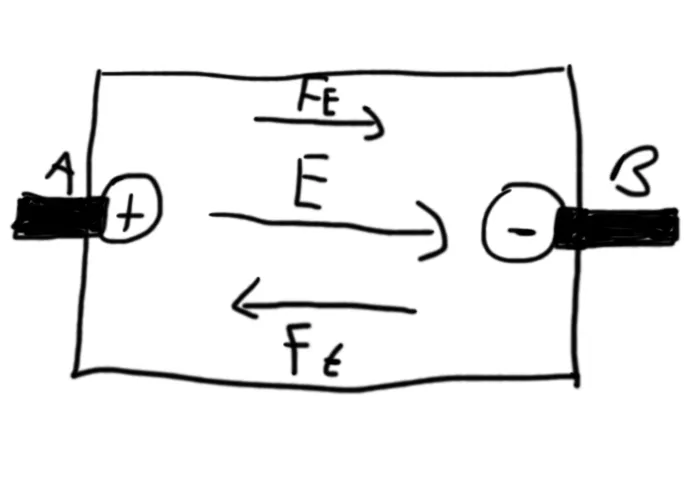The electric field E of course goes from the positive to the negative terminal and so a force in the same direction gets applied to any positive charge. But, as already told before this is the "wrong" direction, cause inside of the EMF component the charges are "forced" to move to the other direction which is B -> A. The force that does this is Fε. When in a open-circuit condition the two forces are equal, else the force Fε must be "stronger" and that exactly defines the electromotive force (EMF) and highest possible voltage that this unit can supply. Note that the work done by that force when going from B to A is W = qε which can easily be proved by using equations we covered in previous posts.

So, finally a closed circuit looks like this:The electric current I is caused by the electric field E which of course goes from the positive to negative terminal. The positive charges are "forced" to continue going in that flow-direction by the EMF that we discussed earlier by applyng a force Fε that goes from negative to positive inside of the unit.

Note that in all that '+' is considered the high potential and '-' the low potential!

The potential difference Vab from a point A to a point B is defined as the work produced by the force Fε on a test charge q that moves from A to B (as we said in my previous post). The EMF ε of a energy source is the acting on the opposite displacement B to A and so for an ideal EMF: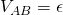Supposing that the wire of a closed circuit has a resistance R we of course know that V = IR which means that:and so when a charge is roaming around the circuit, the increase of ε causes an equal decrease of the potential difference Vab, while the charge moves through a resistor.

Note that we can calculate the electric current I when knowing ε and R.

## Internal resistance

But, of course the EMF unit of a circuit has also some internal resistance which means that the previous formulas are not quite applicable directly! Real energy supplies like Batteries and cells have an internal resistance noted as r and measured in Ohm's (Ω). Supposing this resistance constant of the current inside of the emf-unit of course depends on the potential decrease (voltage drop) V = Ir.

That way the polar voltage inside of the battery is:which is of course smaller then the EMF, because of the term Ir.

We can calculate the maximum electric current I0 that can be produced by using: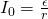The current in the "outside" circuit can still be calculated by the first formula V = IR which means that: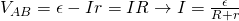and so the current I is equal to the product of the EMF (ε) with the total resistance (R+r) of the circuit. The total resistance is of course a "simple" sum cause the two resistances/resistors are connected one after another (serial connection).

Also worth noting is that the emf is now calculated by: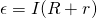which shows us again that the emf is "generating" the current.



Remember that following points:

• The emf force exists in an open circuit, whilst the potential difference only exists in a closed circuit where current flows.
• EMF is the maximum voltage that the energy supply can deliver and the potential difference is always smaller than that maximum possible value of EMF.
• The potential difference depends on the internal resistance, cause it's directly proportional to it, while the EMF doesn't depend on the resistance.



Actual examples and mathematical applications will come in the last post of this "part" of the series and so after covering energy and wattage and maybe even a more advanced post about conductivity of materials!

## REFERENCES:

I'm actually using notes from my study, that I did when learning about this subject 2 years ago and so a lot is not easy to find again from the same sources, where I first searched for it (cause keeping my learning sources was clearly not my concern by that time). But, I tried to find "similar" stuff which is actually quite good and has a lot of what I also have in my notes. Today, I mixed and matched a lot of scientific articles together to make the article contain all that I have in my notes and so here the references for this post:

1. http://farside.ph.utexas.edu -> Figure 17
2. http://www.physicsclassroom.com -> circuit requirements
3. https://en.wikipedia.org -> emf definition
4. https://circuitglobe.com -> definition of emf and potential difference
5. http://physicsnet.co.uk -> internal resistance and formulas

All the mathematical equations that I had in this post where drawn using quicklatex to make them look better!

I will change the previous post section into Electromagnetism-only, cause Classical mechanics can be found in my recap anyway and because it took up half of the article's space!

### Electric fields:

Getting into Electromagnetism -> electromagnetim, electric charge, conductors, insulators, quantization

Coulomb's law with examples -> Coulomb's law, superposition principle, Coulomb constant, how to solve problems, examples

Electric fields and field lines -> Electric fields, Solving problems around Electric fields and field lines

Electric dipoles -> Electric dipole, torque, potential and field

Electric charge and field Exercises -> examples in electric charges and fields

### Electric flux:

Electric flux and Gauss's law -> Electric flux, Gauss's law

Applications of Gauss's law (part 1) -> applying Gauss's law, Gauss applications

Applications of Gauss's law (part 2) -> more Gauss applications

Electric flux exercises -> examples in electric flux and Gauss's law

### Electric potential:

Electric potential energy -> explanation of work-energy, electric potential energy

Calculating electric potentials -> more stuff about potential energy, potential, calculating potentials

Millikan's Oil Drop Experiment -> Millikan's experiment, electronvolt

Cathode ray tubes explained using electric potential -> cathode ray tube explanation

Electric potential exercises (part 1) -> applications of potential

Electric potential exercises (part 2) -> applications of potential gradient, advanced examples

### Capacitance:

Capacitors (Condensers) and Capacitance -> Capacitors, capacitance, calculating capacitance

How to solve problems around Capacitors -> combination, solving problems, simple example

Electric field energy and density -> Electric field energy, energy density

Dielectric materials -> Dielectrics, dielectric constant, permittivity and strength, how to solve problems

Electric capacitance exercises -> examples in capacitance, energy density and dielectrics

### Current, resistance and EMF:

Electric current -> Electric current, current density

Electrical resistivity and conductivity -> Electrical resistivity, conductivity, thermal coefficient of resistivity, hyperconductivity

Electric resistance -> Resistance, temperature, resistors

And this is actually it for today and I hope that you enjoyed it!

Next time I will get into the Power and Wattage of Electronic Circuits!

Bye!

H2
H3
H4
3 columns
2 columns
1 column